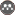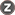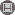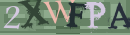Volume 13, Issue 2 (7-2013)                   2013, 13(2): 347-362 | Back to browse issues page

BibTeX | RIS | EndNote | Medlars | ProCite | Reference Manager | RefWorks
Send citation to:Ordokhani Y, Dehestani H. Numerical solution of the nonlinear Fredholm-Volterra-Hammerstein integro-differential equations via Bessel functions. Quarterly Journal of Science Kharazmi University. 2013; 13 (2) :347-362
URL: http://jsci.khu.ac.ir/article-1-1642-en.html
Abstract:   (7358 Views)
In this paper, a collocation method based on the Bessel polynomials is used for the solution of nonlinear Fredholm-Volterra-Hammerstein integro-differential equations (FVHIDEs) under mixed condition. This method of estimating the solution, transforms the nonlinear (FVHIDEs) to matrix equations with the help of Bessel polynomials of the first kind and collocation points. The matrix equations correspond to a system of nonlinear algebraic equations with the unknown Bessel coefficients. Present results and comparisons demonstrate that our estimate has good degree of accuracy and this method is more valid and useful than other methods.In this paper, a collocation method based on the Bessel polynomials is used for the solution of nonlinear Fredholm-Volterra-Hammerstein integro-differential equations (FVHIDEs) under mixed condition. This method of estimating the solution, transforms the nonlinear (FVHIDEs) to matrix equations with the help of Bessel polynomials of the first kind and collocation points. The matrix equations correspond to a system of nonlinear algebraic equations with the unknown Bessel coefficients. Present results and comparisons demonstrate that our estimate has good degree of accuracy and this method is more valid and useful than other methods.
Type of Study: S | Subject: Mathematic
Published: 2013/07/15Rights and permissionsThis work is licensed under a Creative Commons Attribution-NonCommercial 4.0 International License.